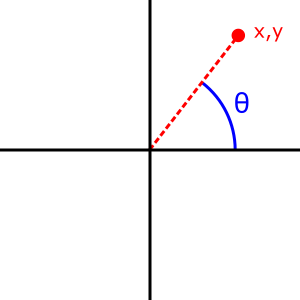# Math.atan2()

The Math.atan2() static method returns the angle in the plane (in radians) between the positive x-axis and the ray from (0, 0) to the point (x, y), for Math.atan2(y, x).

## Syntax

js

Math.atan2(y, x)


### Parameters

y

The y coordinate of the point.

x

The x coordinate of the point.

### Return value

The angle in radians (between -π and π, inclusive) between the positive x-axis and the ray from (0, 0) to the point (x, y).

## Description

The Math.atan2() method measures the counterclockwise angle θ, in radians, between the positive x-axis and the point (x, y). Note that the arguments to this function pass the y-coordinate first and the x-coordinate second.Math.atan2() is passed separate x and y arguments, while Math.atan() is passed the ratio of those two arguments. Math.atan2(y, x) differs from Math.atan(y / x) in the following cases:

In addition, for points in the second and third quadrants (x < 0), Math.atan2() would output an angle less than $-\frac\left\{\pi\right\}\left\{2\right\}$ or greater than $\frac\left\{\pi\right\}\left\{2\right\}$.

Because atan2() is a static method of Math, you always use it as Math.atan2(), rather than as a method of a Math object you created (Math is not a constructor).

## Examples

### Using Math.atan2()

js

Math.atan2(90, 15); // 1.4056476493802699
Math.atan2(15, 90); // 0.16514867741462683


### Difference between Math.atan2(y, x) and Math.atan(y / x)

The following script prints all inputs that produce a difference between Math.atan2(y, x) and Math.atan(y / x).

js

const formattedNumbers = new Map([
[-Math.PI, "-π"],
[(-3 * Math.PI) / 4, "-3π/4"],
[-Math.PI / 2, "-π/2"],
[-Math.PI / 4, "-π/4"],
[Math.PI / 4, "π/4"],
[Math.PI / 2, "π/2"],
[(3 * Math.PI) / 4, "3π/4"],
[Math.PI, "π"],
[-Infinity, "-∞"],
[Infinity, "∞"],
]);

function format(template, ...args) {
return String.raw(
{ raw: template },
...args.map((num) =>
(Object.is(num, -0)
? "-0"
: formattedNumbers.get(num) ?? String(num)
),
);
}

console.log(| x     | y     | atan2 | atan  |
|-------|-------|-------|-------|);

for (const x of [-Infinity, -1, -0, 0, 1, Infinity]) {
for (const y of [-Infinity, -1, -0, 0, 1, Infinity]) {
const atan2 = Math.atan2(y, x);
const atan = Math.atan(y / x);
if (!Object.is(atan2, atan)) {
console.log(format| ${x} |${y} | ${atan2} |${atan} |);
}
}
}


The output is:

| x     | y     | atan2 | atan  |
|-------|-------|-------|-------|
| -∞    | -∞    | -3π/4 | NaN   |
| -∞    | -1    | -π    | 0     |
| -∞    | -0    | -π    | 0     |
| -∞    | 0     | π     | -0    |
| -∞    | 1     | π     | -0    |
| -∞    | ∞     | 3π/4  | NaN   |
| -1    | -∞    | -π/2  | π/2   |
| -1    | -1    | -3π/4 | π/4   |
| -1    | -0    | -π    | 0     |
| -1    | 0     | π     | -0    |
| -1    | 1     | 3π/4  | -π/4  |
| -1    | ∞     | π/2   | -π/2  |
| -0    | -∞    | -π/2  | π/2   |
| -0    | -1    | -π/2  | π/2   |
| -0    | -0    | -π    | NaN   |
| -0    | 0     | π     | NaN   |
| -0    | 1     | π/2   | -π/2  |
| -0    | ∞     | π/2   | -π/2  |
| 0     | -0    | -0    | NaN   |
| 0     | 0     | 0     | NaN   |
| ∞     | -∞    | -π/4  | NaN   |
| ∞     | ∞     | π/4   | NaN   |


## Specifications

Specification
ECMAScript Language Specification
# sec-math.atan2

## Browser compatibility

BCD tables only load in the browser